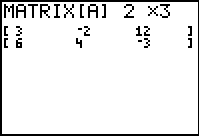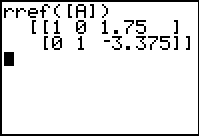# Knowledge Base

## Solution 11990: Solving Simultaneous Equations on a TI-83 Family or TI-84 Plus Family Graphing Calculator.

### How can I solve simultaneous equations on a TI-83 family or TI-84 Plus family graphing calculator?

Simultaneous equations can be solved on a TI-83 family or TI-84 Plus family graphing calculator by entering the coefficients of the equations in a matrix and then using the rref() function. Please Note: The rref() function is in the MATRX, MATH menu.

Example:

Solve for x and y
3x + -2y = 12
6x + 4y = -3

Solution: The equations can also be written as the following matrix:To input and solve the matrix,

1) Press [2nd] [MATRX] (Note: On the TI-83, press [MATRX]).
2) Scroll to Edit.
3) Press  to access matrix A.
4) Input the dimensions  [ENTER]  [ENTER].
5) Input the matrix entries, pressing enter after each value.
6) Press [2nd] [QUIT] [2nd] [MATRX] (Press [2nd] [QUIT] [MATRX] on the TI-83).
7) Scroll to MATH.
8) Press [ALPHA] [B] [2nd] [MATRX]  [)] [ENTER] (With the TI-83, press [ALPHA] [B] [MATRX]  [)] [ENTER]).

The screen will show:Therefore the solution is x = 1.75 and y = -3.375.

Please see the TI-83 family and TI-84 Plus family guidebooks for additional information.

Please Note: The TI-83 family or TI-84 Plus family have the Polynomial Root Finder and Simultaneous Equation Solver App that can be downloaded onto the calculator to provide this feature.